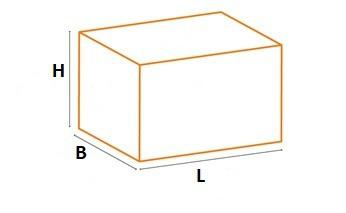﻿ Sky Stock - Volumetric Weight
+6221.2002.2012
example@gmail.com

## Volumetric Weight Calculation

• Light-weight consignments occupying a large space due to dimensions need to be charged as per the volumetric weight calculations as follows:## Domestic Surface Mode:

• Volumetric Weight = LxBxH / 5000 in centimetres

## Domestic Air Mode:

• Volumetric Weight = LxBxH / 6000 in centimetres